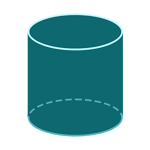Report a Problem
Suggestions

#Calculate The Surface Area of A Right Cylinder

Last updated: Saturday, June 24, 2023
Select a cylindrical shape below
Right Cylinder
Hollow Cylinder
Oblique Cylinder
Right Truncated
Cylindrical Wedge

The surface area of a right cylinder is the total area of all its curved and flat surfaces. It can be calculated using the following formula:

Surface Area = 2πr(h + r)

Where r is the radius of the circular base of the cylinder, and h is its height.

To visualize this, imagine a soda can. The curved part of the can is the lateral surface area, while the top and bottom circular parts are the base areas. To find the total surface area of the can, you would need to add the lateral surface area to the two base areas.

Another example of a right cylinder could be a pipe or a drum. The surface area of the cylinder is important to know in manufacturing, as it helps determine the amount of material needed to construct the cylinder. It is also important in engineering and architecture for calculating the heat transfer, pressure, and fluid dynamics of cylindrical structures.

The formula for determining the surface area of a right cylinder is defined as:
$$SA$$ $$=$$ $$2$$ $$\cdot$$ $$\pi$$ $$\cdot$$ $$r$$ $$\cdot$$ $$(h$$ $$+$$ $$r)$$
$$SA$$: the surface area of the cylinder
$$r$$: the radius of the base
$$h$$: the height of the cylinder
The SI unit of surface area is: $$square \text{ } meter\text{ }(m^2)$$

## Find $$SA$$

Use this calculator to determine the surface area of a cylinder when the radius of the bases and the height are given.
Hold & Drag
CLOSE
$$r$$
$$meter$$
$$h$$
$$meter$$